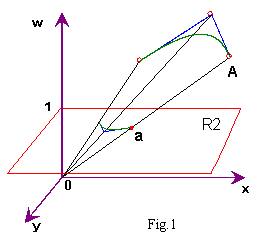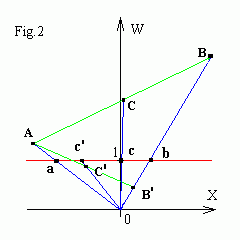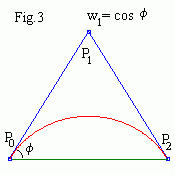# NonUniform Rational B-splines (NURBS)

Recall, that the B-spline is weighted sum of its control points
P(t) = ∑i=0,n Ni,k(t) Pi ,     tk-1 ≤ t ≤ tn+1       (*)
and the weights Ni,k have the "partition of unity" property
i=0,n Ni,k(t) = 1 .
As weights Ni,k depend on the knot vector only, it is useful to add to every control point one more weight wi which can be set independently
P(t) = ∑i=0,n wi Ni,k(t) Pi / ∑i=0,n wi Ni,k(t) .       (**)
Then increasing a weight wi makes the point more influence and attracts the curve to it. The denominator in (**) normalizes weights, so we will get the equation (*) if we set wi = const for all i. Full weights wiNi,k satisfy the "partition of unity" condition again.

Interactive NURBS
Drag the mouse to move a nearest control point (a small blue square in the left window) or change a weight (a small square in the right window colored as corresponding basis function). Drag the mouse with <Shift> to move a knot (a small black square in the right window).

# Perspective projection

Formula (**) has a simple interpretation by perspective projection from 4D space (x, y, z, w). The perspective projection from three dimensions (x, y, w) to two dimensions (the plane R2) is illustrated in Fig.1. A 3D point A is projected along a straight line through the origin to the point of intersection with the plane R2. This is essentially the same process as projecting a 3D object onto a 2D screen with perspective camera. You see in Fig.2, that perspective projection c of a point C = (A+B)/2 is really attracted to b with respect to c' projection of C' = (A+B')/2, as Bw > Aw and B'w < Aw .As since perspective projection of a 4D point (x, y, z, w) has coordinate (x/w, y/w, z/w), therefore formula (**) is the perspective projection of 4D equation
P w(t) = ∑i=0,n Ni,k(t) Piw ,
where Piw is a 4D vector with the components (wixi , wiyi , wizi , wi ) . The perspective projection of Piw is evidently the control point Pi .

4D representation of NURBS is very useful, as since all B-spline's properties are "projected" into rational spline's ones.

There are two different conventions for representing the control points in terms of their 4D coordinates (x,y,z,w):
Homogeneous, in which the coordinates represent the point's position in 4D space. Thus the point's 3D position is (x/w, y/w, z/w).
Weighted Euclidean, in which the coordinates are already considered to have been divided through. Thus the first tree components (x,y,z) directly represent the point's position in 3D space and the fourth w represents its weight.
Weighted Euclidean coordinates are used in interactive nurbs.java applet.

# Conic sections

 Conic section is an intersection of a cone with a plane. The angle at which the plane intersects the cone determines whether the resulting curve is a circle, ellipse, parabola or hyperbola. Conic curves are represented here using quadratic NURBS (n=2, k=3) with the open uniform knots [0 0 0 1 1 1]: parabola (w1 = 1), hyperbola (w1 = 4) and ellipse (w1 = 1/4).

# Circular arcs

The following method can be used to construct a circular arc of quadratic NURBS:
• The legs of the control triangle are of equal length (i.e. it is isosceles).
• The chord connecting the first and the last control points meets each leg at an angle φ equal to half the angular extent of the arc.
• The weight of the inner control point is cos(φ ).
• The open uniform knot vector is [0,0,0,1,1,1].You see below how to get a hole circle with only one NURBS. The knot vectors are [0, 0, 0, 1/3, 1/3, 2/3, 2/3, 1, 1, 1] and [0, 0, 0, 1/4, 1/4, 1/2, 1/2, 3/4, 3/4, 1, 1, 1].

Contents   Previous: Interpolating cubic B-splines   Next: 3D spline curves and surfaces
updated 23 August 2001